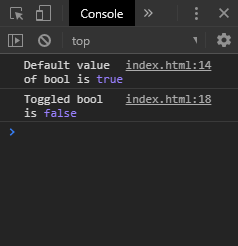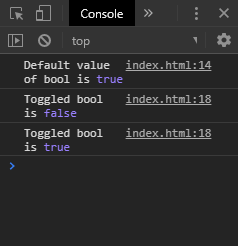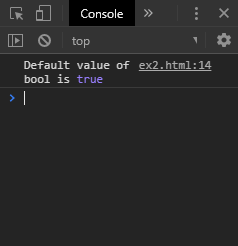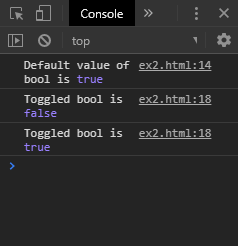GFG App
Open AppBrowser
Continue

# How to toggle a boolean using JavaScript ?

A boolean value can be toggled in JavaScript by using two approaches which are discussed below:

Method 1: Using the logical NOT operator: The logical NOT operator in Boolean algebra is used to negate an expression or value. Using this operator on a true value would return false and using it on a false value would return true. This property can be used to toggle a boolean value. The NOT operator is used before the variable to be toggled and the result is assigned to the same variable. This will effectively toggle the boolean value.

Syntax:

`booleanValue = !booleanValue`

Example:

 `<``html``> ` `<``head``> ` `    ``<``title``> ` `        ``How to toggle a boolean ` `        ``using JavaScript? ` `    `` ` ` ` ` `  `<``body``> ` `    ``<``h1` `style``=``"color: green"``> ` `        ``GeeksforGeeks ` `    `` ` `     `  `    ``<``b``> ` `        ``How to toggle a boolean ` `        ``using JavaScript?  ` `    `` ` `     `  `    ``<``p``> ` `        ``The boolean is toggled whenever ` `        ``the button is pressed. ` `    `` ` `     `  `    ``<``p``> ` `        ``See the console for ` `        ``the output ` `    `` ` ` `  `    ``<``button` `onclick``=``"toggle()"``> ` `        ``Toggle Bool ` `    `` ` `     `  `    ``<``script``> ` `        ``let testBool = true; ` `         `  `        ``console.log('Default value of bool is', ` `                                   ``testBool); ` `     `  `        ``function toggle() { ` `            ``testBool = !testBool; ` `             `  `            ``console.log('Toggled bool is', ` `                                ``testBool); ` `        ``} ` `    `` ` ` ` ` `  ` `

Output:

• Clicking the button once:• Clicking the button twice:Method 2: Using the ternary operator: The ternary operator is used as a shortcut to using the if/else statement. It is used to make a decision based on the condition of expression. It takes three parameters, the condition statement, the expression to be executed if the condition is satisfied and the expression to be executed if the condition is not satisfied.

The boolean value to be toggled is passed as the condition. The expression to be executed if true is given as ‘false’ and expression to be executed if false is given as ‘true’. The result of this operator is assigned back to the boolean value to be toggled. This will effectively toggle the value as a true condition will return false, and a false condition would return true.

Syntax:

`booleanValue = booleanValue? false : true`

Example:

 ` ` `<``html``> ` ` `  `<``head``> ` `    ``<``title``> ` `        ``How to toggle a boolean ` `        ``using JavaScript? ` `    `` ` ` ` ` `  `<``body``> ` `    ``<``h1` `style``=``"color: green"``> ` `        ``GeeksforGeeks ` `    `` ` `     `  `    ``<``b``> ` `        ``How to toggle a boolean ` `        ``using JavaScript?  ` `    `` ` `     `  `    ``<``p``> ` `        ``The boolean is toggled ` `        ``whenever the button is ` `        ``pressed. ` `    `` ` `     `  `    ``<``p``> ` `        ``See the console ` `        ``for the output ` `    `` ` ` `  `    ``<``button` `onclick``=``"toggle()"``> ` `        ``Toggle Bool ` `    `` ` `     `  `    ``<``script``> ` `        ``let testBool = true; ` `        ``console.log('Default value of bool is', ` `                    ``testBool); ` `     `  `        ``function toggle() { ` `            ``testBool = testBool ? false : true; ` `             `  `            ``console.log('Toggled bool is', ` `                                ``testBool); ` `        ``} ` `    `` ` ` ` ` `  ` `

Output:

• Clicking the button once:• Clicking the button twice:My Personal Notes arrow_drop_up